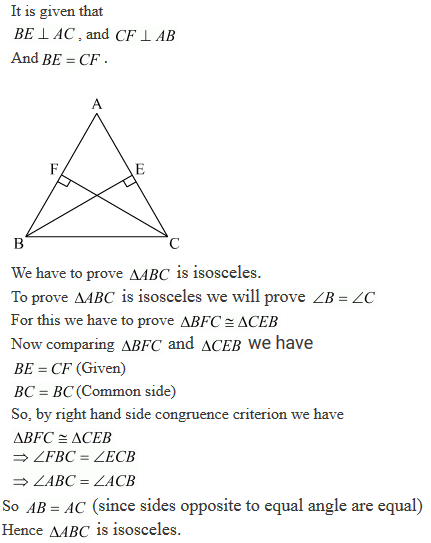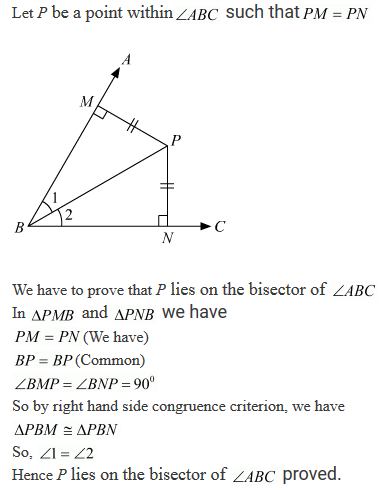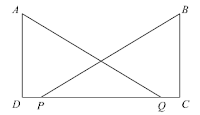#### Chapter 10 Congruent Triangles R.D. Sharma Solutions for Class 9th Exercise 10.5

Exercise 10.5

1.
ABC is a triangle and D is the mid-point of BC. The perpendiculars from D to AB and AC are equal. Prove that the triangle is-isosceles.

Solution2. ABC is a triangle in which BE and CF are, respectively, the perpendiculars to the sides AC and AB. If BE = CF, prove that ΔABC is isosceles.

Solution3. If perpendiculars from any point within an angle on its arms are congruent, prove that it lies on the bisector of that angle.

Solution4. In Fig. 10.99, AD ⊥ CD and CB ⊥ CD. If AQ = BP and DP = CQ, prove that
∠DAQ = ∠CBP.Solution

Given in the fig. 10.99, AD  CD and CB  CD.
And AQ = BP and DP = CQ,
To prove that  DAQ =  CBP
Given that DP = QC
DP + PQ = PQ + QC
DQ = PC ……(i)
Now, consider triangle DAQ and CBP,
We have
ADQ =  BCP = 90°   [given]
AQ = BP    [given]
And DQ = PC     [From (i)]
So, by RHS congruence criterion, we have
ΔDAQ ≅ ΔCBPNow,
DAQ = CBP   [Corresponding parts of congruent triangles are equal]
Hence, proved

5. ABCD is a square, X and Y are points on sides AD and BC respectively such that AY= BX. Prove that BY = AX and ∠BAY = ∠ABX.

Solution

Given that,
ABCD is a square, X and Y are points on sides AD and BC respectively such that AY = BX.
To prove: BY = AX and ∠ BAY = ∠ ABX
Join B and X, A and Y.Since, ABCD is a square
∠ DAB = ∠ CBA = 90°
∠ XAB = ∠ YAB = 90° ……(i)
Now, consider triangle XAB and YBA
We have
∠ XAB = ∠ YBA = 90°.   [From (i)]
BX = AY [given]
And AB = BA [Common side]
So, by RHS congruence criterion.
We have ΔXAB≅ΔYBA
Now, we know that corresponding parts of congruent triangles are equal.
BY = AX and ∠ BAY = ∠ ABX
Hence proved

6. Which of the following statements are true (T) and which are false (F):
(i) Sides opposite to equal angles of a triangle may be unequal.
(ii) Angles opposite to equal sides of a triangle are equal.
(iii) The measure of each angle of an equilateral triangle is 60°.
(iv) If the altitude from one vertex of a triangle bisects the opposite side, then the triangle may be isosceles.
(v) The bisectors of two equal angles of a triangle are equal.
(vi) If the bisector of the vertical angle of a triangle bisects the base, then the triangle may be isosceles.
(vii) The two altitudes corresponding to two equal sides of a triangle need not be equal.
(viii) If any two sides of a right triangle are respectively equal to two sides of other right triangle, then the two triangles are congruent.
(ix) Two right triangles are congruent if hypotenuse and a side of one triangle are respectively equal equal to the hypotenuse and a side of the other triangle.

Solution

(i) False
(ii) True
(iii) True
(iv) False
(v) True
(vi) False
(vii) False
(viii) False
(ix) True

7. Fill in the blanks in the following so that each of the following statements is true.
(i) Sides opposite to equal angles of a triangle are .......
(ii) Angle opposite to equal sides of a triangle are .......
(iii) In an equilateral triangle all angles are ........
(iv) In a ABC if ∠A = ∠C, then AB = ............
(v) If altitudes CE and BF of a triangle ABC are equal, then AB = ........
(vi) In an isosceles triangle ABC with AB = AC, if BD and CE are its altitudes, then BD is ...... CE.
(vii) In right triangles ABC and DEF, if hypotenuse AB = EF and side AC = DE, then ΔABC ≅ Δ .........

Solution

(i) Sides opposite to equal angles of a triangle are equal

(ii) Angles opposite to equal sides of a triangle are equal

(iii) In an equilateral triangle all angles are equal Reason: Since all sides are equal in a equilateral triangle. the angles opposite to equal sides will be equal .

(iv) In a Δ ABC, if ∠ A = ∠ C, then AB = BC

(v) If altitudes CE and BF of a triangle ABC are equal, then AB = AC

(vi) In an isosceles triangle Δ ABC with AB = AC, if BD and CE are its altitudes, then BD is equal to CE

(vii) In right triangles ABC and DEF, if hypotenuse AB = EF and side AC = DE, then, ΔABC=ΔEFD.